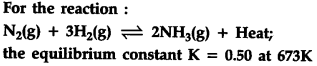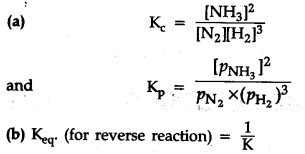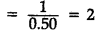# 1.Write the expression for and \${{K}_{p}}\$1.Write the expression for and {{K}_{p}}
2.What will be the equilibrium constant value for the reverse reaction at 673 K ?
3.What is the effect of increasing temperature on the yield of N\${{H}{3}} ? 4.What is the effect of adding {{N}{2}}(g) and {{H}{2}}(g) on the yield of N{{H}{3}} ? 5.What is the effect of increasing pressure on the yield of NH3? Or Classify the following species into Lewis acids and Lewis bases: O{{H}^{-}},{{F}^{-}},{{H}^{+}},B{{F}{3}} Calculate the pH of a solution in which 0.4 g of NaOH is dissolved in water to give 200 mL of solution. 6.{{H}{2}}\$0 can act both as Bronsted arid and base. Write it’s corresponding conjugate base and conjugate arid.© As this is an exothermic reaction, on increasing
the temperature, the reaction will move in reverse direction. This results in decrease in concentration of N\${{H}{3}} (d) On increasing the concentration of {{N}{2}}(g) and {{H}{2}}(g), the yield of N{{H}{3}}\$ will increase.
(e) On increasing the pressure, the reaction will
move in forward reaction in which number of moles of gas or pressure decreases.
OR